# 4500 Dominator Air Cleaner Spacers

### ALLOWS YOU TO RAISE YOUR SCOOP or AIR FILTER# 1/2″ 4500 Spacer \$39.99 + shipping# 3/4″ 4500 Spacer \$39.99 + shipping# 1″ 4500 Spacer \$44.99 + shipping# 2″ 4500 Spacer \$59.99 + shipping

### NOTE:  Only the 1″ spacer will work with very old style Holley Dominators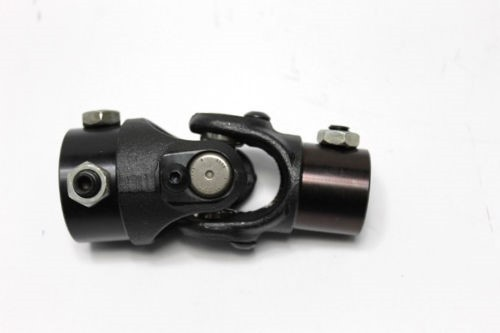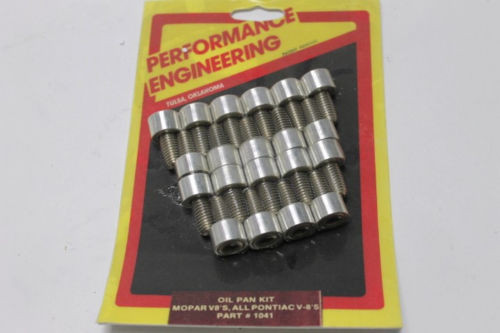# AFCO CHEETA QUICK CHANGE GEAR CHART

http://static.speedwaymotors.com/pdf/Pro%20Gears.pdf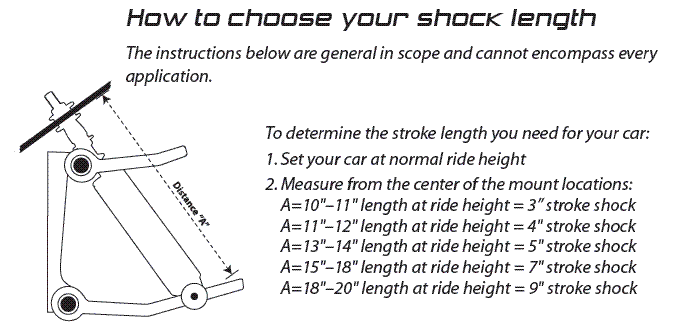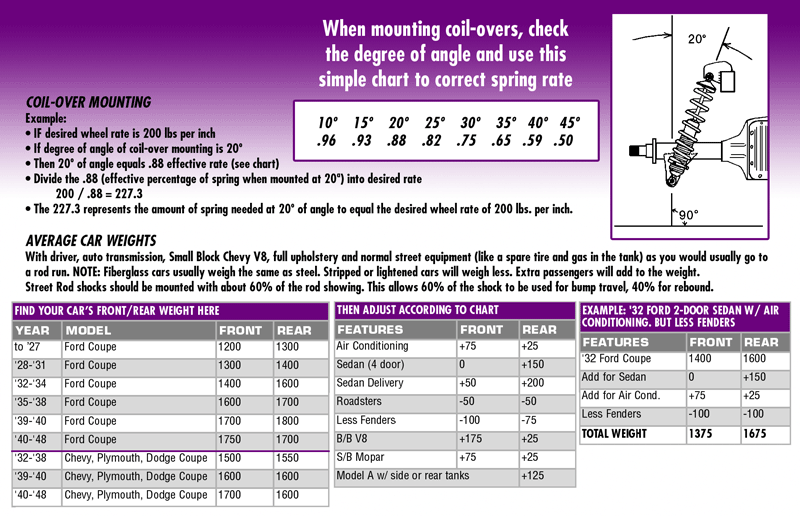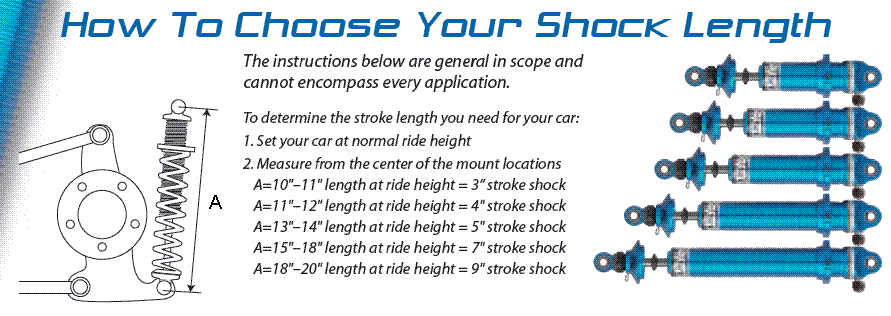# Street Performance & Racing Spring Rate Tech

What is spring rate?
Spring rate refers to the amount of weight that is needed to compress a spring one inch. If the rate of the spring is linear, its rate is not affected by the load that is put on the spring. For example, say you have a 200 lb. per inch spring – it will compress 1” when a 200 lb. load is placed onto the spring. If another 200 lbs. is put onto the spring, the spring will compress another inch. At this point the load on the spring is 400 lbs. The rate of the spring, however, remains constant at 200 lbs. per inch.

Spring Rate Correction for Angle Mounting
If your spring is mounted at an angle you will need to consider that in your spring calculations. Measure the angle of your spring from vertical (A) in degrees. Use the examples provided on this page or the formula below to determine your Angle Correction Factor (ACF).

#ACF= COS/A degree

Shock Angle Angle Correction Factor (ACF)
10° .98
15° .96
20° .94
25° .91
30° .87
35° .82
40° .77
45° .71The greater the installed angle, the stiffer the spring rate must be to support the same weight. First, determine the spring needed for the application if the spring is installed straight up. Then, to compensate for installations at different angles, find your ACF using the chart or formula above, and divide your straight mounted spring rate by your ACF.

Example:
Straight Mounted Spring = 200 lbs.
Spring Mounted at 30° = 200/.87 = 230 lbs.

The 230 lbs. represents the spring rate needed when mounted at a 30° angle to equal the desired spring  rate of 200 lbs. when standing straight up.

How to select the spring rate for independent suspension

Select your spring rate by using the following calculations:D1 = The distance from the pivot point of the a-arm to the mounting point of the spring/shock.
D2 = The distance from the pivot point of the a-arm to the center of the ball joint.
Divide D1 by D2 to calculate the force ratio (Fr).
Force Ratio (Fr) = D1/D2
Weigh your car to determine the weight on the wheels (W).
Divide the weight on the wheel by Fr to determine the force required at the spring (Sf).
W/Fr=Sf

If your spring is mounted at an angle you will need to consider that in your spring calculations. Measure the angle (A) of your spring from vertical in degrees. Use the table above to determine your Angle Correction Factor (ACF). Now divide the Spring Force (Sf) from the earlier calculation by the Angle Correction Factor (ACF) to get the Adjusted Spring Force (ASf).
Sf/ACF=ASf

Note: This calculation determines spring FORCE not spring RATE.

The required Adjusted Spring Force (ASf) can now be used to select the proper spring rate for your application. The required spring force can be obtained several different ways. A lighter rate spring with more preload or a stiffer rate spring with less preload will generate the same spring force. The softer rate will generate a smoother ride while the stiffer spring will result in a firmer ride. You need to consider these options when you are selecting the proper spring rate for your application.

Springs should typically be compressed 25-30% of the free length when supporting the weight of the vehicle. Drag race cars will normally use a lighter rate spring (about 30%) to promote weight transfer while a street car will use a firmer rate spring (about 25%).

ASf/(spring free length x 0.25) = Firmer Spring Rate
Asf/(spring free length x 0.30) = Softer Spring Rate

Spring rate calculations for solid axle suspension are the same as above except the Force Ratio (Fr) = 1.

Here is a Link for a spring chart:

https://www.qa1.net/tech/documents/Spring-Rate-Chart-Vehicle-Weights.pdf